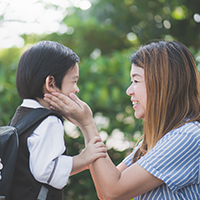# 北京小学奥赛数学希望报名

• 北京小学奥赛数学希望报名。如果平时数学学习比较好，通过初试，能够进入决赛就达到目标，主要是通过竞赛看看自己的能力和掌握的知识还有什么不足。下面，小编给您带来北京小学奥赛数学希望报名的相关内容，供大家参考。

该竞赛活动分两试进行。第一试(每年三月进行)以各地(省、市、县、〔区〕、学校)为单位组织参赛学生，在全国各参赛学校同时进行，各测试点按命题委员会下发的评分标准进行阅卷、评分，从中按七分之一的比例按成绩择优选拔参加第二试的选手。第二试(每年四月进行)由当地《数理天地》编委分会或地、市级教研室或教育学院、教科所、教师进修学校统一组织，测试结束后，各测试点将试卷密封，向组委会挂号寄出，由命题委员会阅卷，从中按八分之一的比例按成绩评定一、二、三等奖，分别授予金、银、铜奖牌及获奖证书。对组织工作做得出色的地区或学校，组委会颁发“希望杯”数学邀请赛组织奖。

日本国算数奥林匹克委员会对此项赛事非常关注，该委员会事务局局长若杉荣二先生专程来华同邀请赛组委会洽谈参赛事宜，并从1996年开始，已连续三年组织日本部分中学生参加了竞赛活动，由此开创了中国社会团体举办同类竞赛走出国门的先例。美国、德国的有关组织也与组委会联系合作事宜。

常用数据及规律

1.圆周率常取数据
3.14×1＝3.14
3.14×2＝6.28
3.14×3＝9.42
3.14×4＝12.56
3.14×5＝15.7
3.15×6＝18.84
3.14×7＝21.98
3.14×8＝25.12
3.14×9＝28.26

2.常用特殊数的乘积
25×3＝75
25×4＝100
25×8＝200
125×3＝375
125×4＝500
125×8＝1000
625×16＝10000
37×3=111

3.常用平方数
11²=121 12²=144 13²=169 14²=196 15²=225 16²=256 17²=289 18²=324 19²=361 10²=100 20²=400 30²=900 40²=1600 50²=2500 60²=3600 770²=4900 80²=6400 15²=225 25²=625 35²=1225 45²=2025 55²=3025 65²=4225 75²=5625 85²=7225

4.关于常用分数与小数的互化
1/2=0.5 4=0.25 3/4=0.75 1/5=0.2 2/5=0.4 3/5=0.6 4/5=0.8 1/8=0.125 3/8=0.375 5/8=0.625 7/8=0.875 1/20=0.05 3/20=0.15 7/20=0.35 9/20=0.45 11/20=0.55 1/25=0.04 2/25=0.08 3/25=0.12 4/25=0.16 6/25=0.24

5.常用立方数
1³=1 2³=8 3³=27 4³=64 5³=125 6³=216 7³=343 8³=512 9³=729

由于篇幅原因，以上只是部分内容。

获取完整版方法：

1. ， _点击领取_“希望”数学1-6年级国际巅峰对决个人战试题及答案、邀请赛历届试题 ，填写姓名、电话信息后即可跳转百度云盘地址，免费获取。

同时点击领取资料_“希望”数学1-6年级国际巅峰对决个人战试题及答案，点击链接 或者下方图片即可领取！

这一期的北京小学奥赛数学希望报名小编就介绍到这里，学习数学，针对不同类型的题目，我们可以用各种各样的方法，在练习中要根据实际情况选择正确的方法，就会省时省力地完成题目。有任何疑问请拨打学而思爱智康免费咨询电话：400-810-2620！

小编推荐：

23686名爸妈正在学而思・爱智康讨论，马上加入->03:54

•北京小升初

•北京幼升小

•幼儿园入园

•更多帮### 家长帮